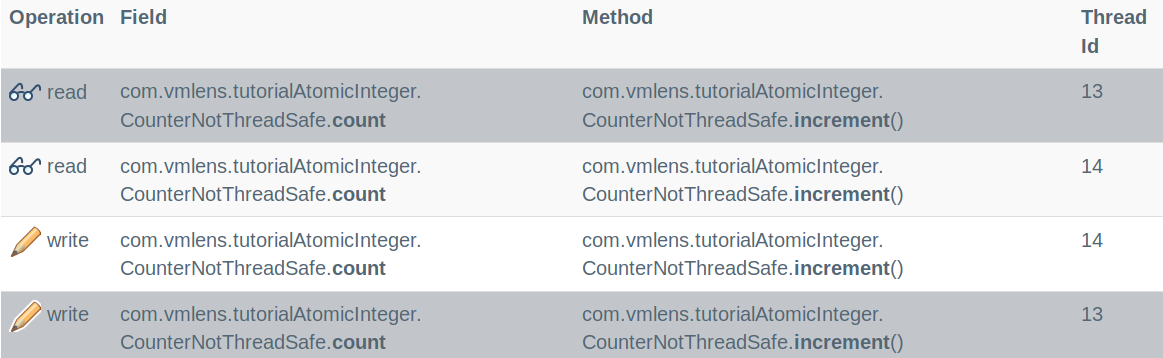{{announcement.body}}
{{announcement.title}}

# Java Concurrency: AtomicInteger

DZone 's Guide to

# Java Concurrency: AtomicInteger

· Java Zone ·
Free Resource

Comment (0)

Save
{{ articles.views | formatCount}} Views

`AtomicInteger` is a class specially designed to update integers in a thread-safe way. Why do we need this a class? Why can we not simply use a volatile int? And how can we use `AtomicInteger`?

## Why AtomicInteger?

The following shows an example of a not thread-safe counter using a `volatile int`:

``````public class CounterNotThreadSafe {
private volatile int count = 0;
public void increment() {
count++;
}
public int getCount() {
return count;
}
}``````

You can download the source code of all examples from GitHub here.

We store the count in the volatile int count, line 2. We need the volatile keyword to make sure that the threads always see the current values, as explained in greater detail here. We increment the counter by using the `++` operation, line 4. To check if the class is thread-safe, we use the following test:

``````public class ConcurrencyTestCounter {
@Interleave
private void increment() {
counter.increment();
}
@Test
public void testCounter() throws InterruptedException {
Thread first = new Thread( () ->    {  increment();  } ) ;
Thread second = new Thread( () ->   {  increment();  } ) ;
first.start();
second.start();
first.join();
second.join();
assertEquals( 2 , counter.getCount());
}
}``````

To test if the counter is thread-safe, we need two threads, created in line 9 and 10. We start those two threads, line 11 and 12. And then, we wait until both are ended using thread `join`, line 13 and 14. After both threads are stopped, we check if the count is two, line 15.

To test all thread interleavings, we use the annotation `Interleave`, line 3, from vmlens. The `Interleave` annotation tells `vmlens` to test all thread interleavings for the annotated method. Running the test, we see the following error:

``ConcurrencyTestCounter.testCounter:22 expected:<2> but was:<1>``

The reason for the error is that since the operation `++` is not atomic the two threads can override the result of the other thread. We can see this in the report from `vmlens`:In the case of the error, both threads first read the variable count in parallel. And then, both write to the variable. This leads to the wrong value 1.

To fix this bug, we use the class `AtomicInteger`:

``````public class CounterUsingIncrement {
private final AtomicInteger count = new AtomicInteger();
public  void increment() {
count.incrementAndGet();
}
public int getCount() {
return count.get();
}
}``````

Instead of using an `int` we use `AtomicInteger` for the variable count, line 2. And instead of using the operation `++`, we use the method`incrementAndGet`, line 4.

Now, since the method `incrementAndGet` is atomic, e.g. the other thread always sees the value either before or after the method call, the threads can not override the value of their calculation. So, the count is now always 2, for all thread interleavings.

## How to Use AtomicInteger

The class `AtomicInteger` has multiple methods that allow us to update the `AtomicInteger` atomically. For example, the method `incrementAndGet` atomically increments the `AtomicInteger` and `decrementAndGet` decrements the `AtomicInteger`.

But the method `compareAndSet` is special. This method allows us to implement arbitrary calculations atomically. The `compareAndSet` method takes two parameters, the expected current value, and the new value. The method atomically checks if the current value equals the expected value. If yes, the method updates the value to the new value and return true. If not, the method leaves the current value unchanged and returns false.

The idea to use this method is to let `compareAndSet` check if the current value was changed by another thread while we calculated the new value. If not we can safely update the current value. Otherwise, we need to recalculate the new value with the changed current value.

The following example shows how to use the `compareAndSet` to implement our counter:

``````public  void increment() {
int current = count.get();
int newValue = current + 1;
while( ! count.compareAndSet( current , newValue ) ) {
current = count.get();
newValue = current + 1;
}
}``````

We first read the current value, line 2. Then, we calculate the new value, line 3. And then, we check using `compareAndSet` if another thread changed the current value, line 4. `compareAndSet` will update the current value if the current value is unchanged and return true. Otherwise, if the value was changed `compareAndSet` will return false. Since this test might fail multiple times, we need to use a while loop. If the value was changed by another thread, we need to get the current changed value, line 5. And then recalculate the new value, line 6 and try to update again.

## Conclusion

`AtomicInteger` lets us update integers in a thread-safe way. Use atomic methods like `incrementAndGet` or `decrementAndGet` for simple types of calculations. And use the methods get and `compareAndSet` for all other types of calculations.

Topics:

Comment (0)

Save
{{ articles.views | formatCount}} Views

Published at DZone with permission of Thomas Krieger , DZone MVB. See the original article here.

Opinions expressed by DZone contributors are their own.